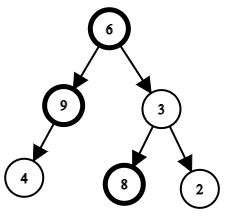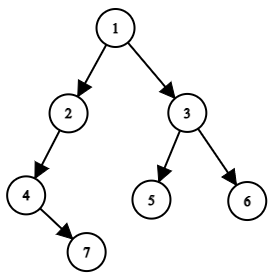# Largest Tree Value

Posted: 12 Mar, 2021
Difficulty: Moderate

## PROBLEM STATEMENT

#### You have been given the ‘ROOT’ of a binary tree having ‘N’ nodes, you need to find the largest value in each row or level of the binary tree.

##### For Example``````For the above binary tree,
Max value at level 0 = 6.
Max value at level 1 = max(9 , 3) = 9
Max value at level 2 = max (4, 8, 2) = 8
``````
##### Input Format:
``````The first line contains an integer 'T' which denotes the number of test cases.

The first line of each test case contains elements of the tree in the level order form. The line consists of values of nodes separated by a single space. In case a node is null, we take -1 in its place.
````````````For example, the input for the tree depicted in the above  image would be :
1
2 3
4 -1 5 6
-1 7 -1 -1 -1 -1
-1 -1
``````

#### Explanation :

``````Level 1 :
The root node of the tree is 1

Level 2 :
Left child of 1 = 2
Right child of 1 = 3

Level 3 :
Left child of 2 = 4
Right child of 2 = null (-1)
Left child of 3 = 5
Right child of 3 = 6

Level 4 :
Left child of 4 = null (-1)
Right child of 4 = 7
Left child of 5 = null (-1)
Right child of 5 = null (-1)
Left child of 6 = null (-1)
Right child of 6 = null (-1)

Level 5 :
Left child of 7 = null (-1)
Right child of 7 = null (-1)
``````
##### Note :
``````The above format was just to provide clarity on how the input is formed for a given tree.
The sequence will be put together in a single line separated by a single space. Hence, for the above-depicted tree, the input will be given as:

1 2 3 4 -1 5 6 -1 7 -1 -1 -1 -1 -1 -1
``````
##### Output Format
``````For each test, Print the maximum value at each row of the binary tree.

Print the output of each test case in a separate line.
``````
##### Note:
``````You do not need to print anything, it has already been taken care of. Just implement the given function.
``````
##### Constraints:
``````1 <= T <= 50
0 <= N <= 10^3
1 <= DATA <= 10^4

'DATA' is the value of the binary tree node.

Time Limit: 1 sec
``````Approach 1

Approach:

1. The main idea is to do a depth-first search with a slight modification.
2. We will recursively traverse the tree for its left and right child.
3. We will maintain an extra variable ‘LEVEL’ which will keep the level number of the elements and a ‘RESULT’ vector where ‘RESULT[i]’ will store the maximum value at level ‘i‘.
4. If the value of the current ‘LEVEL’ is equal to the size of elements in the ‘RESULT’ vector that means we have encountered the element at this level first time. So we will push the current element to ‘RESULT’.
5. Otherwise, we will compare the current element with the ‘RESULT [ LEVEL ]’. If the current element is larger than 'RESULT [ LEVEL ]', we will update 'RESULT [ LEVEL ]' = current element.
6. In the end, the ‘RESULT’ vector will contain the maximum values at each tree row.

Algorithm:

1. If the root is NULL we will simply return.
2. Otherwise, compare the current ‘LEVEL’ with the size of the ‘RESULT’ vector.
• If level = size of ��RESULT’
• Push root -> DATA to ‘RESULT’.
• Else
• ‘RESULT [ LEVEL ]’ = max( RESULT [ LEVEL ] , root -> DATA)
3. Recursively call for left and right children for next level i.e ( root -> LEFT , LEVEL + 1) and (root - > RIGHT, LEVEL +1).